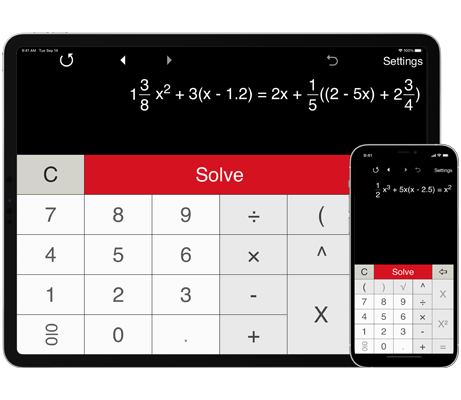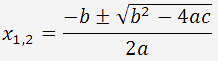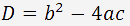A handy calculator with steps to solve quadratic equations with real and complex roots.
Supports pre-defined formats for complete and incomplete quadratic equations.
Shows steps.

## Calculator for solving quadratic equations step by step

This is an easy to use quadratic equation calculator with steps to solve quadratic equations (or second degree equations) in the form ax2 + bx + c = 0, ax2 + bx = 0 and ax2 + c = 0.

To find the roots of a quadratic equation (or 2nd degree equation), enter the coefficients 'a', 'b' and 'c' and click 'Solve'.
'a', 'b' and 'c' are real numbers with a ≠ 0. Quadratic Equation Solver supports whole, fractional and decimal coefficients.

To solve incomplete quadratic equations, you can select the pre-defined formats in the Settings window.
To solve a quadratic equation without a linear term,select the ax2 + c = 0 format.
To solve a quadratic equation without a constant term, select the ax2 + bx = 0 format.

Quadratic equation solver lets you view your recent calculations. With the Back and Forward buttons you can check or recall recent calculations.

Quadratic Equation Solver solves quadratic equations (2nd degree equations) with real and complex roots.

Quadratic Equation Solver calculates the discriminant and the roots of a quadratic equation using the quadratic formula.The discriminant could be positive, zero or negative. Depending on the value of discriminant, there are three possibilities:

If the discriminant is positive (D > 0), the equation has two real roots.

If the discriminant is zero (D = 0), there is only one real root.

If the discriminant is negative (D < 0), there are no real roots - the equation has two complex roots.